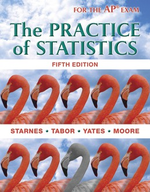### Solution Found!# Analyzing Teen Texting: Confidence Interval & Plausibility

Chapter 8, Problem 36

(choose chapter or problem)

Get Unlimited Answers! Check out our subscriptions
QUESTION:

A Pew Internet and American Life Project survey found that 392 of 799 randomly selected teens reported texting with their friends every day.

(a) Calculate and interpret a 95% confidence interval for the population proportion $$p$$ that would report texting with their friends every day.

(b) Is it plausible that the true proportion of American teens who text with their friends every day is 0.45? Use your result from part (a) to support your answer.

##### Analyzing Teen Texting: Confidence Interval & PlausibilityWant To Learn More? To watch the entire video and ALL of the videos in the series:

Discover how to calculate the 95% confidence interval for a population proportion using sample data. Through a practical example about texting habits among American teens, learn the implications of this statistical tool and how to assess plausibility.

QUESTION:

A Pew Internet and American Life Project survey found that 392 of 799 randomly selected teens reported texting with their friends every day.

(a) Calculate and interpret a 95% confidence interval for the population proportion $$p$$ that would report texting with their friends every day.

(b) Is it plausible that the true proportion of American teens who text with their friends every day is 0.45? Use your result from part (a) to support your answer.

Step 1 of 3

(a) It is required to estimate the population proportion p of teens who reported texting with their friends daily with 95% confidence. Let $$p$$ be the sample proportion of teens who reported texting with their friends every day, as written below:

$$\begin{array}{l} p=\frac{392}{799} \\ p=0.49 \end{array}$$

PLAN: To use a one-sample z-interval for $$p$$, the conditions are checked below:

1. Random: The data of teens have been selected randomly. 10% condition: The sample size n has a value of 799 is less than 10% of the population of all teens.

2. Large counts: The expression can be calculated as,

\begin{aligned} n p & =799 \times 0.49 \\ & \approx 392 \\ & \geq 10 \\ n(1-p) & =799 \times(1-0.49) \\ & \approx 407 \\ & \geq 10 \end{aligned}